# Engine pulley

The engine has a 1460 rev / min (RPM). Disc diameter is 350 mm. What will be the disc peripheral speed in RPM? Pulleys on the engine has diameter 80mm, on a disc has diameter 160mm.

Result

n2 =  730 1/min
n3 =  13.378 m/s

#### Solution:Leave us a comment of example and its solution (i.e. if it is still somewhat unclear...):

Showing 0 comments:Be the first to comment!#### To solve this example are needed these knowledge from mathematics:

Do you want to convert length units?

## Next similar examples:

1. Pulley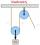On wheels with a diameter of 40 cm is fixed rope with the load. Calculate how far is load lifted when the wheel turns 7 times?
2. Wheel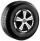What is the wheel diameter if on the 0.38 km track turns 128 times?
3. Tree shadow 3What is the diameter of a tree around which is 3m 9dm 7cm long rope wrapped? How big is its cross-sectional area?
4. MineWheel in traction tower has a diameter 5 m. How many meters will perform an elevator cabin if wheel rotates in the same direction 49 times?
5. Ping time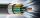Calculate theoretical ping time between Orlando and Shenzhen which is 14102 km distant. Ping time measures the round-trip time for small messages sent from the origin to a destination that is echoed back to the source. The name comes from active sonar term
6. Wheel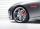How many times turns the wheel of a passenger car in one second if car run at speed 100 km/h. Wheel diameter is d = 62 cm.
7. Bicycle wheelBicycle wheel has a diameter of 70 cm. Approximately how many times the wheel rotates at 4.7 km long trip?
8. BaseBase of building is circle with diameter 25 m. Calculate the circumference of a circular trench witch diameter is 41 cm wider than the diameter of the base.
9. FlowerbedIn the park there is a large circular flowerbed with a diameter of 12 m. Jakub circulated him ten times and the smaller Vojtoseven times. How many meters each went by and how many meters did Jakub run more than Vojta?
10. Circle r,DCalculate the diameter and radius of the circle if it has length 52.45 cm.
11. Saw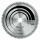Imagine that the saw blade with diameter 40 cm has one tooth colored with a different color. How long is path describing by the tip of the tooth after 15 minutes, if the blade is rotated 13365 times?
12. CircleWhat is the radius of the circle whose perimeter is 6 cm?
13. Equator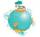Suppose that tourist went on foot over the Globe equator. How many meters more track made ​​his hat on his head as the shoes on your feet? The radius of the earth is 6378 km and height of the tourist is 1.7 m.
14. Clock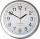How long is trajectory of second hand of hours for day, if is 15 mm long?
15. Feet to milesA student runs 2640 feet. If the student runs an additional 7920 feet, how many total miles does the student run?
16. Two gears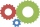The two gears fit together. The larger gear has 32 teeth, the smaller has 20 teeth less. How many times does turn a smaller gear if the bigger gear turns three times?
17. Scale of the mapDetermine the scale of the map if the actual distance between A and B is 720 km and distance on the map is 20 cm.